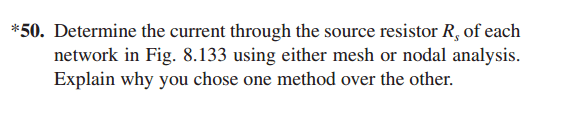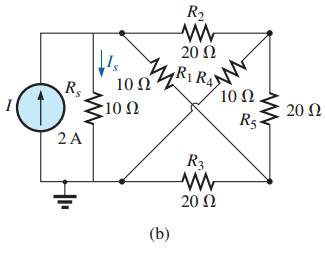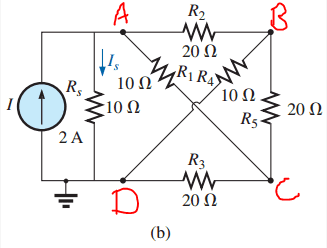# Problem with circuit analysis

• Engineering
hello guys

i have been trying to solve this problem for a long time but i couldn't so i need your help..iam trying to analyze the circuit using mesh analysis

so i converted the current so to voltage source E=2*10=20V

i don't know how to redraw the circuit taking this shape ..so can you help me please ??

Thanks GuysI can see four nodes in this circuit. Mark those nodes as A, B, C, D starting from the top in clockwise direction. like this:Now redraw the circuit by first marking the nodes A, B, C, D one below the other. Then connect the components between the nodes in such a way that you get a planar network. Now write down the equations by mesh analysis.

But if you observe the circuit again, you will see that they have already grounded node D. So the simpler way is: Nodal analysis!

Whenever i see a non planar circuit, I blindly proceed using nodal analysis.

Hope this helpedYou can follow this method to redraw any circuit.

(1) Mark all the nodes using notations of your choice.
(2) Then start redrawing by marking these nodes in a different manner such that, when you connect the components back, you obtain a planar circuit!
(3) If you find two or more nodes such that they are equipotential (Like in a balanced wheatstone's bridge), mark both the nodes with a common notation and then redraw the circuit.

•1 person
The Electrician
Gold Member
Simply move R1. Instead of as it is, going from upper left to lower right, starting at the upper left node, go up and clockwise around the upper right node, down to the lower right node.

In other words, instead of connecting R1 "inside the square", go around the "outside" of the square.

•1 person
thx guys this helped me alot :)